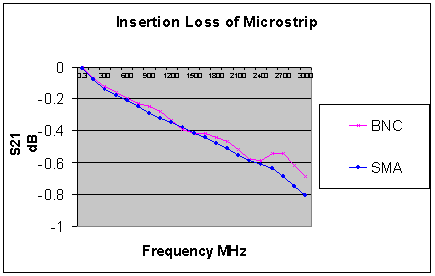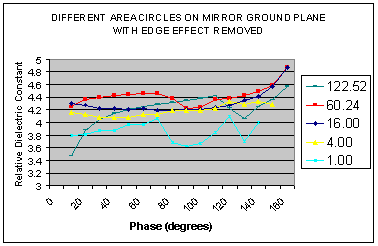# Measuring Relative Dielectric Constant of PCB's Using Parallel Plate Method and a Vector Network Analyser page 4

Figure 7Continuing the study with larger Sample Sizes

Larger boards were made with single circles from a single sheet of material in order to find the optimum size for a sample. The circular capacitor method was used to calculate the εr and the results are shown in Figure 8.

Figure 8.The two larger circles (248.17 and 122.52 square cm) do not coincide with the other two samples at 900. They are clearly too large but they do give some useful clues as to the method of selecting the optimum size, which will be discussed later.

Confirmation of Measurement Point

It is possible to confirm that the 900 point is the best measurement point, as can be seen from Figure 9 which includes some of the earlier results with smaller circles.

Figure 9.It can be seen in Figure 9 that the circles of 60.24 cm2 and 4.00 cm2, coincide at 900 only. They are the largest and smallest possible sample sizes for the PCB material and only the result at 900 can be relied upon.

1  2  3  4  5  >  Page

Technical Article Index